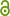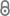# Derivative-Free Multiobjective Trust Region Descent Method Using Radial Basis Function Surrogate Models

M.B. Berkemeier, S. Peitz, Mathematical and Computational Applications 26 (2021).https://www.mdpi.com/2297-8747/26/2/31/pdf
Journal Article | Published | English
Abstract
We present a flexible trust region descend algorithm for unconstrained and convexly constrained multiobjective optimization problems. It is targeted at heterogeneous and expensive problems, i.e., problems that have at least one objective function that is computationally expensive. The method is derivative-free in the sense that neither need derivative information be available for the expensive objectives nor are gradients approximated using repeated function evaluations as is the case in finite-difference methods. Instead, a multiobjective trust region approach is used that works similarly to its well-known scalar pendants. Local surrogate models constructed from evaluation data of the true objective functions are employed to compute possible descent directions. In contrast to existing multiobjective trust region algorithms, these surrogates are not polynomial but carefully constructed radial basis function networks. This has the important advantage that the number of data points scales linearly with the parameter space dimension. The local models qualify as fully linear and the corresponding general scalar framework is adapted for problems with multiple objectives. Convergence to Pareto critical points is proven and numerical examples illustrate our findings.
Publishing Year
Journal Title
Mathematical and Computational Applications
Volume
26
Issue
2
Article Number
31
eISSN
LibreCat-ID

### Cite this

Berkemeier MB, Peitz S. Derivative-Free Multiobjective Trust Region Descent Method Using Radial  Basis Function Surrogate Models. Mathematical and Computational Applications. 2021;26(2). doi:10.3390/mca26020031
Berkemeier, M. B., & Peitz, S. (2021). Derivative-Free Multiobjective Trust Region Descent Method Using Radial  Basis Function Surrogate Models. Mathematical and Computational Applications, 26(2). https://doi.org/10.3390/mca26020031
@article{Berkemeier_Peitz_2021, title={Derivative-Free Multiobjective Trust Region Descent Method Using Radial  Basis Function Surrogate Models}, volume={26}, DOI={10.3390/mca26020031}, number={231}, journal={Mathematical and Computational Applications}, author={Berkemeier, Manuel Bastian and Peitz, Sebastian}, year={2021} }
Berkemeier, Manuel Bastian, and Sebastian Peitz. “Derivative-Free Multiobjective Trust Region Descent Method Using Radial  Basis Function Surrogate Models.” Mathematical and Computational Applications 26, no. 2 (2021). https://doi.org/10.3390/mca26020031.
M. B. Berkemeier and S. Peitz, “Derivative-Free Multiobjective Trust Region Descent Method Using Radial  Basis Function Surrogate Models,” Mathematical and Computational Applications, vol. 26, no. 2, 2021.
Berkemeier, Manuel Bastian, and Sebastian Peitz. “Derivative-Free Multiobjective Trust Region Descent Method Using Radial  Basis Function Surrogate Models.” Mathematical and Computational Applications, vol. 26, no. 2, 31, 2021, doi:10.3390/mca26020031.
All files available under the following license(s):Closed Access